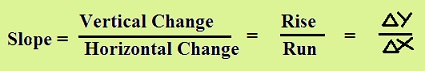In analytical geometry, we use algebraic formulas to calculate geometrical measures. Slope is primarily defined as a simple concept to explain many mathematical, physical and commercial phenomena.

The slope or gradient of a straight line is a measure of its steepness.The slope of a line is the ratio of vertical change to horizontal change between any two points lying on the straight line. The vertical and horizontal changes are often called the Rise and the Run. Generally, Rise and Run are correspondingly represented by $\bigtriangleup$y and $\bigtriangleup$x.Slope of a Line Formula

The slope m of a line containing any two points P(x1, y1) and P(x2, y2) is given as follows:

m = $\frac{\text{Change in y coordinates}}{\text{Change in x coordinates}}$ = $\frac{y_{2} - y_{1}}{x_{2} - x_{1}}$ provided x2 - x1 $\neq$ 0.

Suppose, we have the change in y coordinates = - 4 and the change in x coordinates = 3, then the slope of the line is - $\frac{4}{3}$. This means the line falls 4 units vertically for every 3 units across.

A line which rises from left to right on the coordinate plane has a positive slope, while a line which falls from left to right has a negative slope. The slope of a horizontal line is zero, as there is no change in the y coordinates of the points lying on the line and y1 = y2 => y2 - y1 is hence equal to zero.

All points on a vertical line have the same x coordinate. Thus, x1 = x2 and x2 - x1 = 0. Since division by zero is undefined, the slope of a vertical line is undefined.

How to find the Slope of a Line?

It is straightforward to find the slope of a line, if the coordinates of two points are given. We can just plug the coordinates in the formula and calculate it.

Let us find the slope of a line passing through (2, 3) and (-5, 4).
Thus, the slope of the line m = $\frac{y_{2} - y_{1}}{x_{2} - x_{1}}$ = $\frac{4 - 3}{-5 - 2}$ = - $\frac{1}{7}$

If the equation of a line is given, we can identify the slope by writing the equation in slope intercept form, that is in the form y = mx + b.
Suppose the equation of a line is given as 2x + y = 5.
Rewriting the equation in slope intercept form, we get y = -2x + 5, where m = - 2.
Hence, the slope of the line = - 2.

When the graph of a line is given and we need to read the coordinates of two points, then we can determine the slope using the slope formula.

Let us find the slope of line whose graph is given below:Two points on the line are identified as (2, 3) and (0, -4).
Using the slope formula, m = $\frac{y_{2}-y_{1}}{x_{2}-x_{1}}$

= $\frac{-4-3}{0-2}$

= $\frac{-7}{-2}$

= 3.5

Slope Formula Examples

Given below are some of the examples in slope formula.

Solved Examples

Question 1: Find the slope of the line, whose x and y intercepts are correspondingly, 5 and -3.
Solution:
The coordinates of the x and y intercepts are (5, 0) and (0, -3).
Using the slope formula, m = $\frac{y_{2}-y_{1}}{x_{2}-x_{1}}$

= $\frac{-3-0}{0-5}$

= $\frac{-3}{-5}$

= $\frac{3}{5}$

This means the line rises 3 units up for every 5 units across.

Question 2: Prove that the slopes of the two diagonals of a square are +1 and -1.Solution:
The two adjacent sides of the square can be taken along the coordinate axes as shown in the diagram.
The vertices of the square are hence O (0, 0), A(0, a) , B (a, a) and C (a, 0), where a is the length of the side of the square.

Using slope formula, m = $\frac{y_{2}-y_{1}}{x_{2}-x_{1}}$

Slope of the diagonal OB = $\frac{a-0}{a-0}$ = $\frac{a}{a}$ = 1

Slope of the diagonal AC = $\frac{0-a}{a-0}$ = $\frac{-a}{a}$ = -1